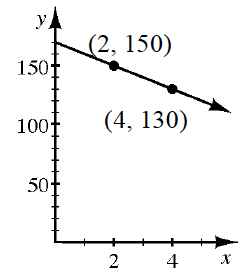### Home > CCA > Chapter 5 > Lesson 5.2.1 > Problem5-54

5-54.

Draw a slope triangle and use it to find the equation of the line shown in the graph below. Homework Help ✎Use the same method you used in problem 5-45.

$y=−10x+170$